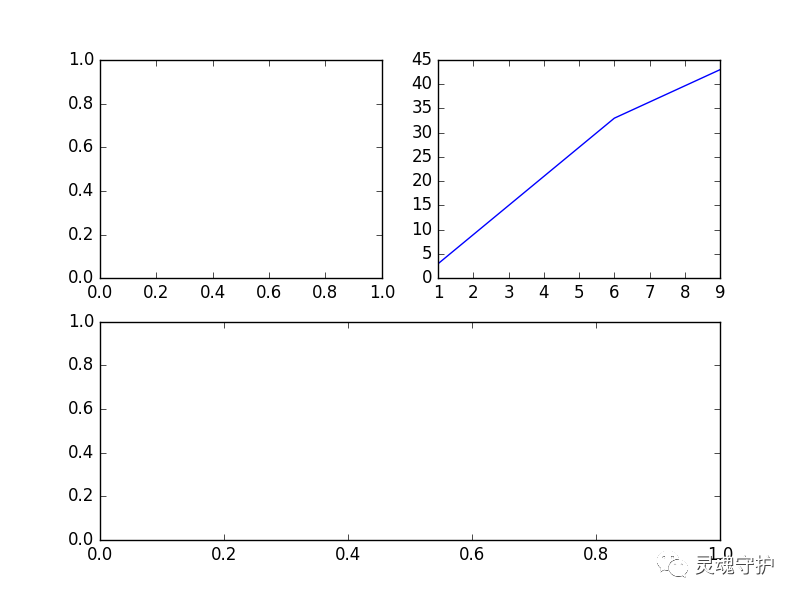python大数据（二）

matplotlib 数据可视化分析

from matplotlib import  pylab
import numpy# 下面2行定义X轴，Y轴x=[1,2,3,4,8]
y=[1,2,3,4,8]# plot的方法是这样使用（x轴数据,y轴数据,展现形式）pylab.plot(x,y)   # 先把x,y轴的信息塞入pylab里面，再调用show方法来画图pylab.show()    # 这一步开始画图,默认是至线图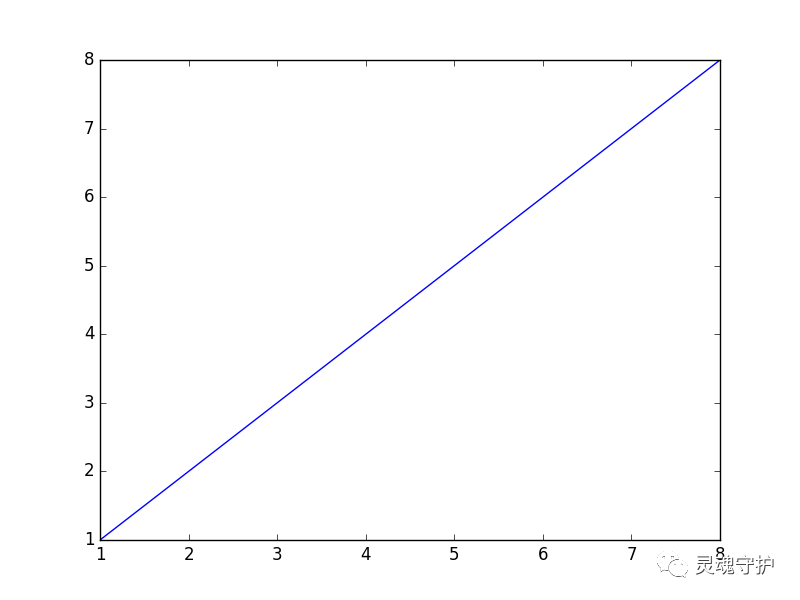下面说说修改图的样式

1. 直线图（默认）

2. - 直线

3. -- 虚线

4. -. -.形式

5. : 细小虚线

1. c-青色

2. r-红色

3. m-品红

4. g-绿色

5. b-蓝色

6. y-黄色

7. k-黑色

8. w-白色

关于形状，有下面几种：
1. s 方形

2. * 星形

3. p 五角形

pylab.plot(x,y,'or')   # 添加O表示画散点图，r表示redpylab.show()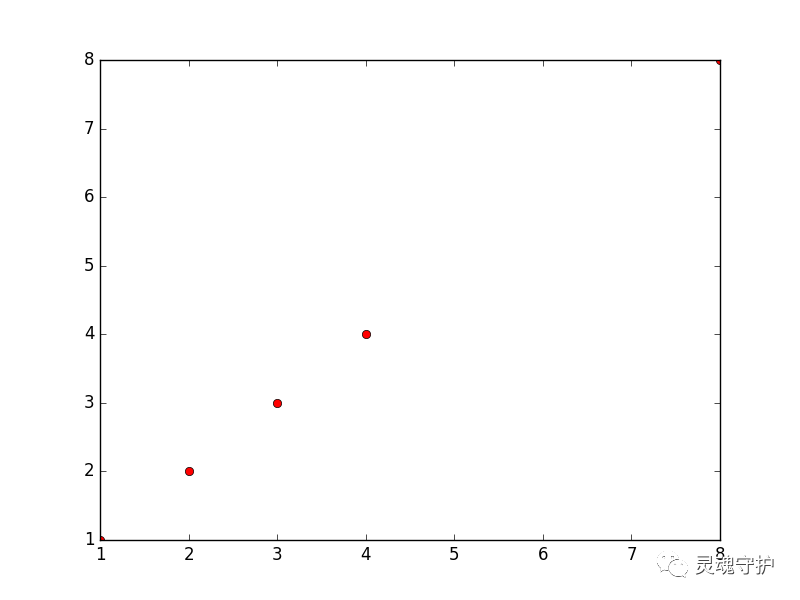pylab.plot(x,y,'r:')pylab.show()

pylab.plot(x,y,'pr--')   #p是图形为五角星，r为红色，--表示虚线pylab.title('for learnning')   # 图形标题pylab.xlabel('args')   # x轴标签pylab.ylabel('salary')   # y轴标签pylab.xlim(2)    # 从y轴的2开始做线pylab.show()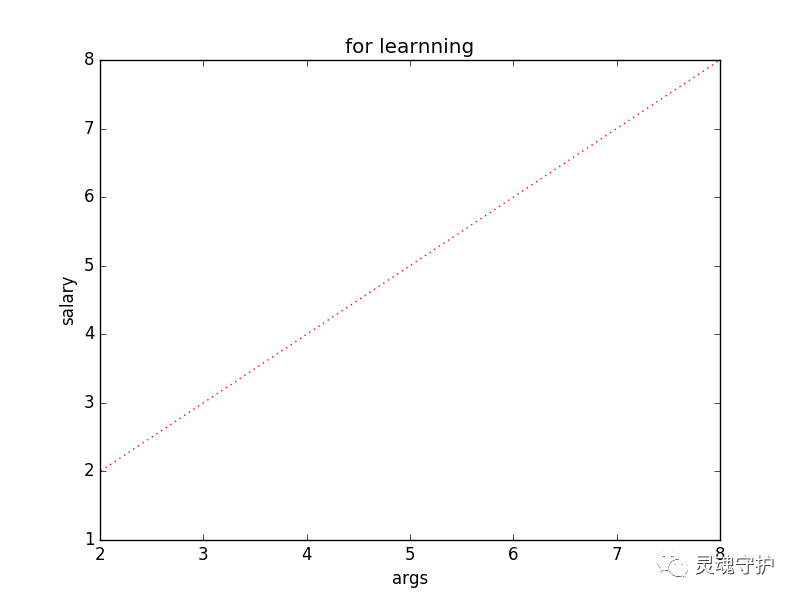直方图

data1 = numpy.random.normal(5.0,4.0,10)   # 正态随机数pylab.hist(data1)
pylab.show()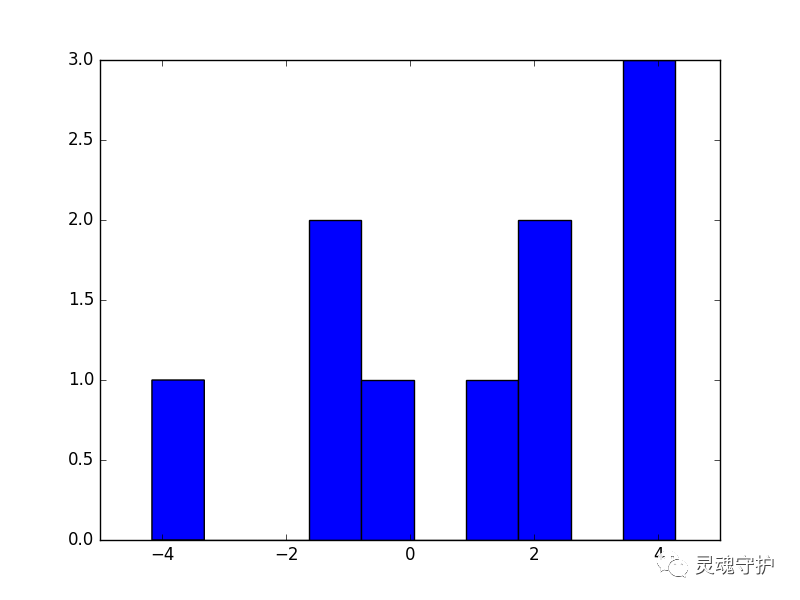Y轴为出现的次数，X轴为这个数的值（或者是范围）

还可以指定直方图类型通过histtype参数：

1. bar ：is a traditional bar-type histogram. If multiple data
are given the bars are aranged side by side.

2. barstacked ：is a bar-type histogram where multiple
data are stacked on top of each other.

3. step ：generates a lineplot that is by default
unfilled.

4. stepfilled ：generates a lineplot that is by default
filled.

sty=numpy.arange(1,30,2)
pylab.hist(data1,histtype='stepfilled')
pylab.show()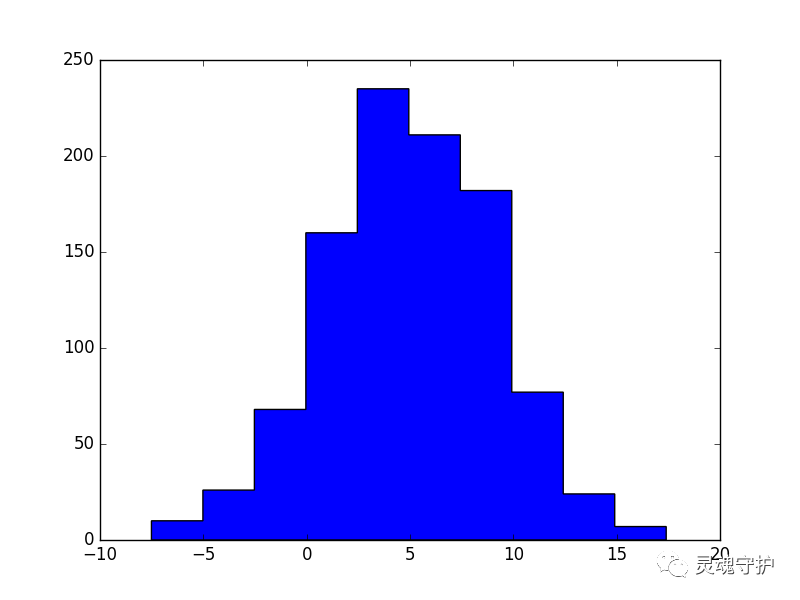子图功能

#subplot(行,列,当前区域)pylab.subplot(2,2,1)   # 申明一个大图里面划分成4块（即2*2），子图使用第一个区域（坐标为x=1,y=1）pylab.subplot(2,2,2)  # 申明一个大图里面划分成4块（即2*2），子图使用第二个区域（坐标为x=2,y=2）x1=[1,4,6,9]
x2=[3,21,33,43]
pylab.plot(x1,x2)   # 这个plot表示把x,y轴数据塞入前一个子图中。我们可以在每一个子图后使用plot来塞入x,y轴的数据pylab.subplot(2,1,2)  # 申明一个大图里面划分成2块（即），子图使用第二个区域（坐标为x=1,y=2）pylab.show()